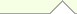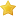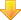Computer Science Books
 Artificial IntelligenceCompiler DesignComputation TheoryComputer AlgorithmComputer ArchitectureComputer GraphicsFunctional ProgrammingInformation TheoryNumerical ComputationOOD/OOPOperating SystemProgramming TheoryHome About Us Link to us Sitemap Contact Us Post QueriesA Computational Introduction to Number Theory and Algebra (V. Shoup)RatingsDownload / View bookA Computational Introduction to Number Theory and Algebra (V. Shoup)
by

File Type :Online
Number of Pages :NA

Description
This book introduces the basic concepts from computational number theory and algebra, including all the necessary mathematical background. Covered topics are: Basic properties of the integers, Congruences, Computing with large integers, Euclid�s algorithm, The distribution of primes, Abelian groups, Rings, Finite and discrete probability distributions, Probabilistic algorithms, Probabilistic primality testing, Finding generators and discrete logarithms in Zp, Quadratic reciprocity and computing modular square roots, Modules and vector spaces, Matrices, Subexponential-time discrete logarithms and factoring, Polynomial arithmetic and applications.Sponsored linksSimilar BooksHome  |  About Us  |  Sitemap  |  Contact Us  |  Link to us  |  Post Your Queries |